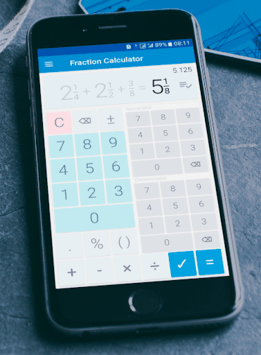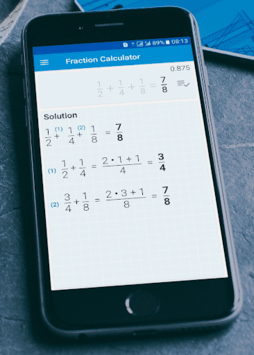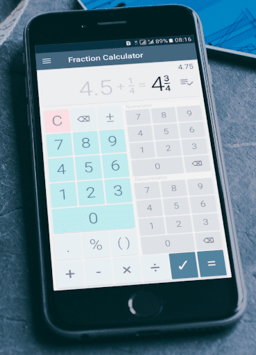# Fractions Calculator - detailed solution available APK

👍👍 Fraction calculator with detailed solution. Perfect fraction problem solver

### APK Details

 Category Education Last Updated 2018-03-12 Latest Version 2.4 Size 5.12 MB Installations 1000000+ Android version 4.1 and up Content Rating Everyone

### Screenshots## Fractions Calculator - detailed solution available APK Description

Best and free offline fraction calculator ✌, supporting also decimals, percentages, and parentheses that shows a detailed solution for all calculations. 😊

At school 🏫 or home 🏠 need to solve simple or complex math fraction problems, convert fractions into decimals, or decimals into fractions? - this fraction calculator will do it ✅ and will show how it was done 😺

Perfect for students, parents, teachers, and woodworkers to solve or verify fraction everyday problems.

Features of this fraction calculator free app ➕ ➖ ❌ ➗

🍉 Supports combining decimal and fractional numbers. Automatically reduces fraction result to its simplest form.

🍇 Converts fractions into decimals and decimals into fractions. Calculated result is displayed using fractions. Equivalent value in decimals is also available.

🍌 Shows a detailed solution, step by step for all fraction problems and calculations.

🍎 History of previous calculations is available.

🍒 Besides basic math operations such as add, subtract, multiply and divide; fraction calculator allows calculating percentages for fractions.

🍍 Works with big numbers. No limits 😋

We have made this fraction calculator app available in multiple colors 🌈 for you!